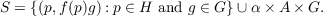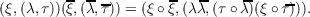#### Vol. 43, No. 1, 1972

 Download this articleFor screen For printingRecent Issues Vol. 325: 1  2 Vol. 324: 1  2 Vol. 323: 1  2 Vol. 322: 1  2 Vol. 321: 1  2 Vol. 320: 1  2 Vol. 319: 1  2 Vol. 318: 1  2Online Archive Volume: Issue:The Journal Subscriptions Editorial Board Officers Contacts Submission Guidelines Submission Form Policies for Authors ISSN: 1945-5844 (e-only) ISSN: 0030-8730 (print) Special Issues Author Index To Appear Other MSP Journals
Automorphisms on cylindrical semigroups

### Joan Geramita

Vol. 43 (1972), No. 1, 93–105
##### Abstract

This paper characterizes the automorphisms of a cylindrical semigroup S in terms of the automorphisms of the defining subgroups and subsemigroups. The following theorem is representative of the type of information given in this paper.

Let F : R A be a dense homomorphism of the additive real numbers to the compact abelian group A. Let λ be a positive real number. Multiplication by λ shall also denote the automorphism of A whose restriction to F(R) is given by FλF1. The set of all such λ for a given F is called Λ1Γ.

Theorem. Let f and λ be as above. Let G be a compact group. LetThen α;S S is an automorphism if and only if α(p,f(p),g) = (λp,f(λp)(f(p))ξ(g));α(,a,g) = (,λa,τ(a)ξ(g)), where τ : A G is a homomorphism into the centre of G and, ξ : G G is an automorphism. Theorem. Let S be as in theorem above. Let 𝒜(G) be the automorphism group of G, and Z(G), the center of G. The automorphism group of S is isomorphic as an abstract group to 𝒜(G) × (AF × Hom(A,Z(G))) with the following multiplicationPrimary: 22A15
##### Milestones